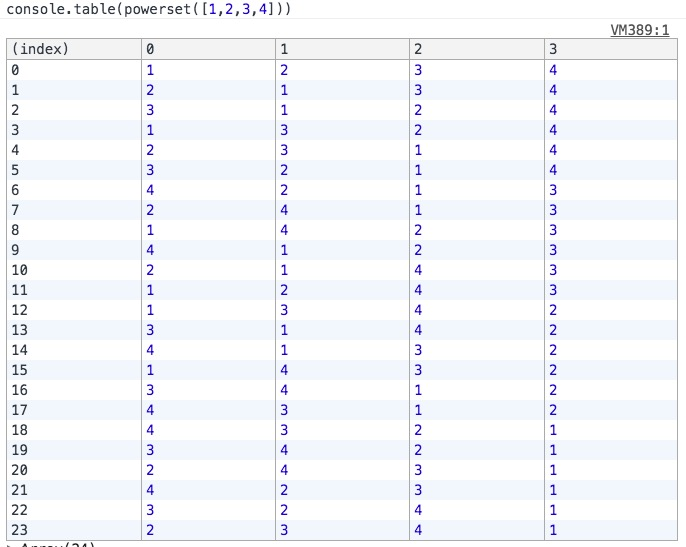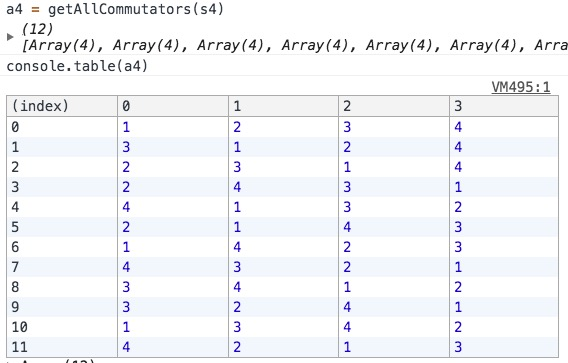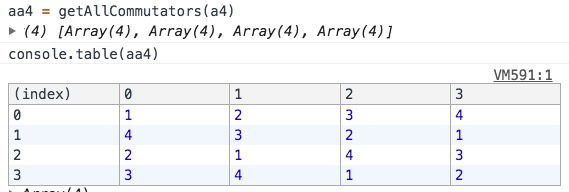# 一般一元五次（及以上）方程无求根公式的直观解释

## 复数的几何意义

Q：为什么要引入复数，难道就不能在实数范围内好好玩耍吗。。
A：还真不能，后面会给出原因。

`(a, b)`

「加法」： `(a, b) + (c, d) = (a+c, b+d)`
「乘法」： `(a, b) · (c, d) = (ac-bd, ad+bc)`

Q：为啥要定义这么稀奇古怪的结构和运算？
A：别急，后面会有说明。

`(1, 1) · (2, 1) = (1*2-1*1, 1*1+1*2) = (1, 3)`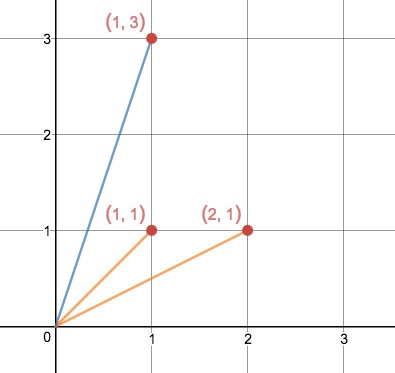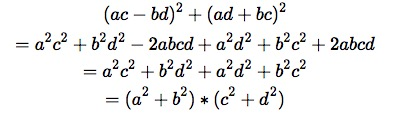`(1, 1) · (1, 1) = (0, 2)`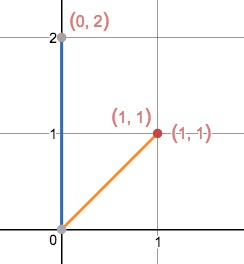Q：可是，这些东西又有什么用？
A：把上述所有形如 `(a, b)` 的结构全部换成 `a + bi`，恭喜你，你已经理解了复数的几何意义

• 复数的加法就是向量的加法
• 复数的乘法得出的结果所代表的向量，其模长等于两乘数的模长之积，其与实数轴的夹角等于两乘数与实数轴的夹角之和。

`1 + 1 = 2` 对应 `(1, 0) + (1, 0) = (2, 0)`
`2 * 3 = 6` 对应 `(2, 0) · (3, 0) = (6-0, 0+0) = (6, 0)`

而且，我们定义的运算同样满足分配律和结合律！感兴趣的读者可以拿纸笔检验一下。

## 根号对复数的作用

√2 到底代表什么意思？

• 它代表的向量与实数轴的夹角的两倍为 0（或 2π 的整数倍）
• 它代表的向量的模长的平方等于 (2^2+0^2)^(1/2) = 2

1 的四分之一次方在复数域是什么意思？

• 向量的模长等于 1
• 向量与实数轴的夹角的 4 倍为 2π 的整数倍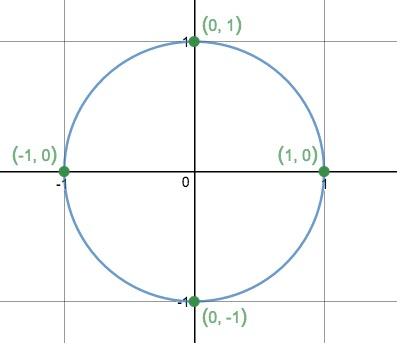• 这些数落在以圆心为原点的某个圆上，这个圆的半径的 n 次方等于 a 的模长
• 这些数所代表的向量与实数轴之间的夹角的 n 倍等于数 a 所代表的向量夹角（加上 2π 的整数倍）
• 可以很容易就得出一个推论：这些数把这个圆的圆周分成 n 等份

## 根的置换

「置换」： 位置的变换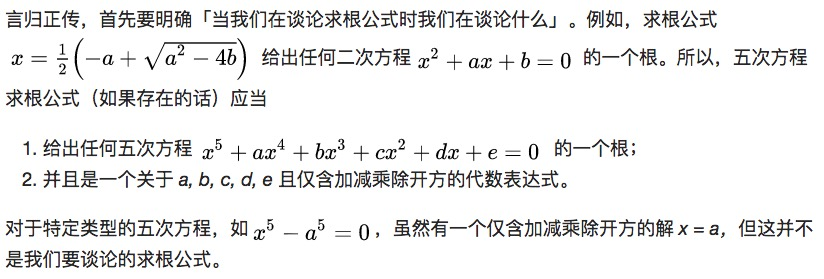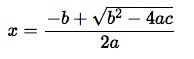x^2 + dx + e = 0 的方程。所以我们只需要考虑

`x^2 + dx + e = 0`

0 = (x - x1)(x - x2)
= x^2 - (x1 + x2)x + x1x2
= x^2 + dx + e

d = -(x1 + x2)
e = x1 * x2

• 方程的根只和方程左边多项式的系数有关
• 方程左边多项式的系数只和方程的根有关

……等等，这不是死循环了吗。。

Excited! 我们已经掌握理解这个定理的钥匙了！

• 方程的求根公式只和方程左边的多项式系数有关
• 这些系数在方程根的置换下保持不变

Q: 我们先来思考这样一个问题：如果方程的求根公式不准用根号，会发生什么？
A: 如果不用根号，那么一个表达式只能求出一个值，也就是说一个求根公式只能得出一个固定的值（点）。

• x1 由这个方程的系数唯一确定
• 这个方程的系数在方程根的置换下保持不变

于是几乎立刻就能得出矛盾：

## 根号的魔法

（由于我们只关心根号的作用，所以下面右侧的图暗含的求根公式为 (b^2 - 4ac)^(1/2) ）

Excited! Excellent! Extraordinary！

## 根号的局限

``````1 -> 2
2 -> 3
3 -> 4
4 -> 5
5 -> 1
``````

``````5 <- 1
1 <- 2
2 <- 3
3 <- 4
4 <- 5
``````

``````1 -> 2 -> 1
2 -> 3 -> 2
3 -> 4 -> 3
4 -> 5 -> 4
5 -> 1 -> 5
``````

• 移动左侧的根（根的置换）
• 右侧求出的根没有发生任何变化
矛盾。

• 置换左侧的根
• 右侧的根也发生了置换

• 置换左侧的根
• 右侧的根依然不发生任何变化

P 2 5 3 4 1 不变
Q 3 4 5 2 1 蓝点顺时针转1/5圈
-P 5 1 3 4 2 不变
-Q 5 4 1 2 3 蓝点逆时针转1/5圈

• 根的置换下多项式系数保持不变
• 求根公式由多项式系数和各种运算（加减乘除和开方）组成
• 不含根号的求根公式只能得出某个唯一的值（即多项式系数的加减乘除运算在根的任意置换下保持不变）
• 开方运算在交换子的作用下保持不变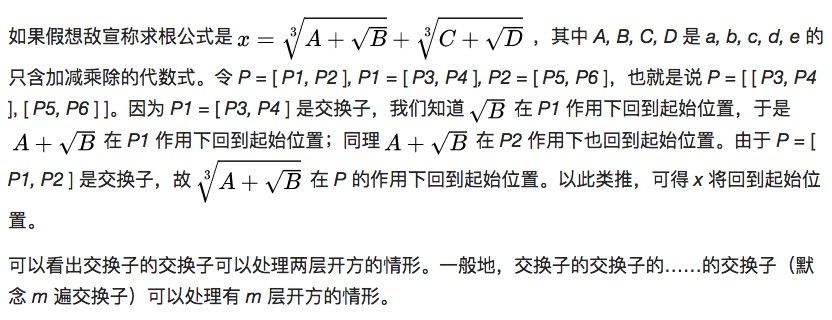## 程序证明

• 置换左侧的根
• 右侧的根依然不发生任何变化

• 根的置换不改变多项式系数
• 每一层交换子可以剥开一层根号（使这层根号的运算保持不变）

``````var powerset = xs => xs.reduceRight((a, x) => a.concat(a.map(y => y.concat(x))), [[]])

function compose (a, b) {
return Array(a.length).fill().map((v, i) => b[a[i] - 1])
}

function invert (a) {
return Array(a.length).fill().map((v, i) => a.indexOf(i + 1) + 1)
}

function commutate (a, b) {
return compose(compose(compose(a, b), invert(a)), invert(b))
}

function getAllCommutators (a) {
var result = []
for (let p1 of a) {
for (let p2 of a) {
result.push(commutate(p1, p2).join('-'))
}
}
result = Array.from(new Set(result))
return result.map(v => v.split('-').map(vv => parseInt(vv)))
}

var s5 = powerset([1, 2, 3, 4, 5]) // 所有置换
var a5 = getAllCommutators(s5) // 所有交换子的结果
var commutatorsOfA5 = getAllCommutators(a5) // 所有交换子的交换子的结果
var t = a5.map(v => v.join('')).sort()
var t2 = commutatorsOfA5.map(v => v.join('')).sort()
console.log(t.join('-') === t2.join('-')) // true, which proved a5 equals to commutatorsOfA5
// 最终我们证明了「所有交换子结果组成的集合」等价于「所有交换子的交换子的结果组成的集合」
``````

## 一元 N 次方程

4 的阶乘等于 24，不算很大的计算量。